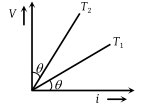The V-i graph for a conductor at temperature T1 and T2 are as shown in the figure. $\left({T}_{2}-{T}_{1}\right)$ is proportional to(1) $\mathrm{cos}2\theta$

(2) $\mathrm{sin}\theta$

(3) $\mathrm{cot}2\theta$

(4) $\mathrm{tan}\theta$